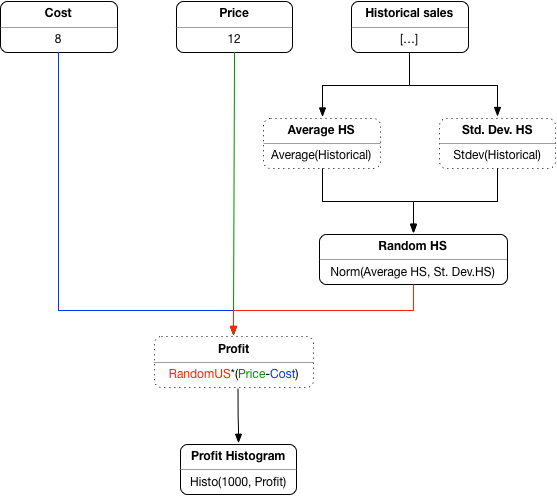## Tuesday, 6 March 2018

### Copying vs. sharing in functional languages

Debugging and profiling functional programs is a challenge, for at least two reasons.

The first reason is that functional programs have no obvious step-by-step flow of control as you would find in imperative programs -- there is no semicolon to induce explicitly a notion of "step". The flow of control is induced implicitly by the evaluation rules of the underlying lambda calculus, for example call-by-need in Haskell, or call-by-value (function-then-argument) in SML, or call-by-value mixing function-then-argument and argument-then-function in OCaml.

The second reason is that the memory footprint of a functional program is much more complex due to automatic copying of arguments and the creation of closures in the heap. Complex memory operations have a crucial impact on the efficiency of functional programs.

Debugging (and profiling) functional languages is also problematic because a low-level (assembly or bytecode) level of debugging is rather useless. Unlike in imperative languages, the conceptual gap between the functional language and the underlying concrete machine is too broad for such a representation to work. A lower-level, yet comprehensible, view of functional computation can be given by so-called 'abstract machines'.

Together with my student Koko Muroya, we have developed a new abstract machine for functional languages based on J.Y. Girard's "Geometry of Interaction" semantics of linear logic proofs. The idea of the machine is to represent the functional program as a graph, which is traversed by a token representing the flow of control. While the token traverses the graph it also causes the graph to reduce by rewriting. This machine is cost-accurate in the sense that it represents faithfully both the number of steps taken during the execution (for a particular evaluation strategy) and the memory footprint. Using a diagrammatic (2D) rather than textual (1D) representation is a convenient tool for describing the memory accurately, since it naturally distinguishes between shared and copied occurrences.

This, for example, is how the program (fun x -> x + x) 1 is represented:
We can observe the syntactically obvious elements of the graph (application "@", function "fun", addition "+", constant "1"). The function-definition subgraph (the lambda expression) is indicated by a dashed yellow line indicating that the subgraph is "copyable" only as a whole. What is distinctive about the GOI-based representation is the representation of variables as edges ("x") which can be shared via special nodes (in the diagram the sharing node is unlabelled). Also distinctive is the representation of abstraction as the connection of the abstracted variables to the fun (lambda) node.

Here is a step-by-step execution in this model. The red arrow indicates the flow of control, pointing initially at the root node of the program, which is the function application.

As we are in a call-by-value language, we are going to evaluate the argument first, which is a reasonable assumption -- evaluating the function first is also possible but more complex and less efficient in many cases. The flow of control advances to the constant "1" and then returns.

After evaluating the argument, the function is inspected next, in three steps. First the flow of control reaches the function, then it "opens" the function, then it enters the function.

At this stage the flow of control is aware that it has visited an application node "@" followed immediately by a function node "fun". This is a combination that can be reduced, by removing these nodes and linking the argument "1" directly to the edge corresponding to the variable "x":
The expression now is equivalent to "1+1", but such that the "1" is actually shared, not replicated (more like let x = 1 in x + x). But the rules of the language evaluation strategy, along with the further propagation of the flow of control will eventually lead to the replication of the argument:
The resulting graph is simply the syntactic tree of a simple expression, which is evaluated to the obvious constant value, in 5 extra stages, of which the last two are:
To avoid being overwhelmed by detail, in a more complex graph we may choose to hide the inside of functions which are not in the process of being evaluated. So, before evaluation the term above would be actually displayed as
Here is now a slightly more complex example in which we can see how closures are created and how they share information in the heap, namely during the execution of the term

let f = (fun x y -> x + y) (0 + 1) in (f 2) + (f 3)

The initial graph is
And here is the stage after a closure has been created via the partial function application (notice "x=1" capture by the closure):

Another interesting stage is the instantiation of the closure twice in the two applications, noting that the argument "x=1" is still shared between the two closures:

Now here is the execution visualiser in action on two more substantial examples, insert-sort vs. bubble-sort, and insert-sort vs. merge-sort. In both cases (sorting small lists) insert-sort wins. We can see how utterly horrible bubble-sort is for functional programming, noting the large amount of copying that happens in this program. We can also see how the constant-factor overheads for merge-sort are too large to be overcome by the asymptotic growth on such a small list.

The visualiser itself will be released soon -- watch this space. It has been implemented by Birmingham University Computer Science student Jack Hughes, as a final year project.

The graph-reduction theory behind this visualiser can be read in The Dynamic Geometry of Interaction Machine: A Token-Guided Graph Rewriter.